# Trigonometry: College Precalculus Lesson Plans Chapter Exam

Exam Instructions:

Choose your answers to the questions and click 'Next' to see the next set of questions. You can skip questions if you would like and come back to them later with the yellow "Go To First Skipped Question" button. When you have completed the practice exam, a green submit button will appear. Click it to see your results. Good luck!

### Page 1

#### Question 3 3. What is the value of cos(θ) given that the equation below is true?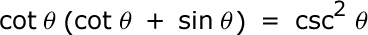#### Question 5 5. Solve the following trigonometric equation for x.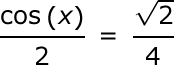### Page 2

#### Question 9 9. In the pictured triangle, ∠A is 137 degrees and ∠B is 28 degrees. If side b is 71 units long, approximately how long is side a?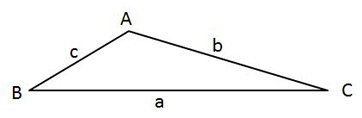#### Question 10 10. Solve the following equation for θ.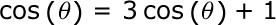### Page 3

#### Question 11 11. What is the function for the following graph?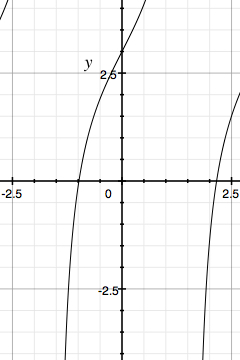#### Question 13 13. What is the following radian measure in degrees?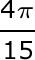#### Question 15 15. Convert the following measurement in radian to degrees.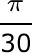### Page 4

#### Question 16 16. Simplify the following expression. Report the answer in degrees.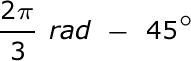#### Question 20 20. In the pictured triangle, side a is 23, side b is 18, and side c is 16. What is the approximate measure of ∠C? When calculating the inverse cosine, round the initial value to the nearest whole number.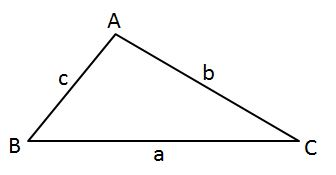### Page 5

#### Question 22 22. What's the value of sec2 (θ) given that the equation below is true?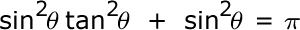#### Question 25 25. In the pictured triangle, side a is 17 and side c is 20. If ∠B is 19 degrees, then approximately how long is side b? Round the value of the cosine to the nearest whole number.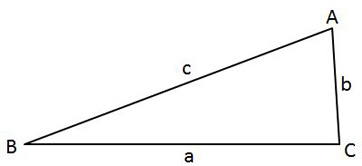### Page 6

#### Question 26 26. What's the value of 2sin(θ)cos(θ) given that the equation below is true?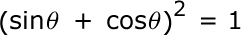#### Question 27 27. In the pictured triangle, side a is 9 and side b is 6. If ∠C is 26 degrees, then approximately how long is side c? Round the value of the cosine to the nearest whole number.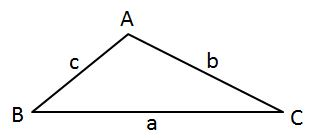#### Question 29 29. Which tangent function would result in the graph below?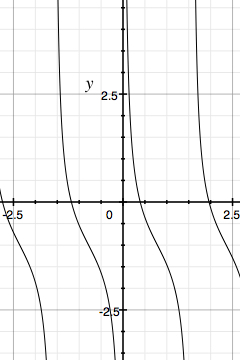#### Trigonometry: College Precalculus Lesson Plans Chapter Exam Instructions

Choose your answers to the questions and click 'Next' to see the next set of questions. You can skip questions if you would like and come back to them later with the yellow "Go To First Skipped Question" button. When you have completed the practice exam, a green submit button will appear. Click it to see your results. Good luck!

Support